Thursday, 18 April 2019 08:04

## The Hyperspherical Lensing of a Glome in Azimuthal Projection. Featured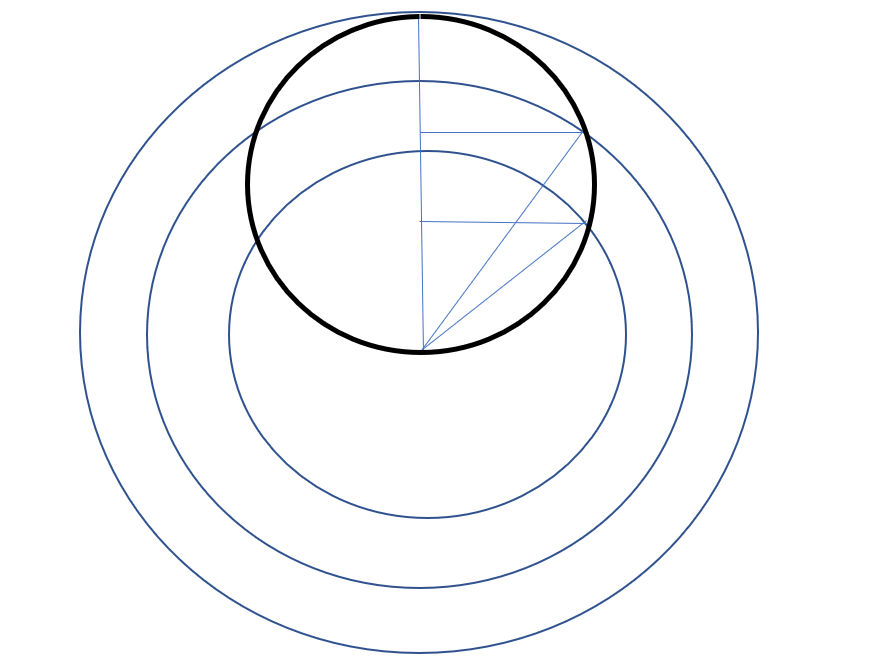Heavy Circle = Hypersphere (Glome), Lighter Circles = Azimuthal Circles

Vertical Line  from centre = Glome antipode distance L, let d = astronomical distance/L.

Horizontals = Glome radii = sqrt(d-d^2)

Hypotenuses = Azimuthal radii = = sqrt(d^2 + (d -d^2))

*Hypotenuses/Horizontals = sqrt(d^2 + (d-d^2)) /sqrt(d-d^2)

*This ratio submits to the following simplification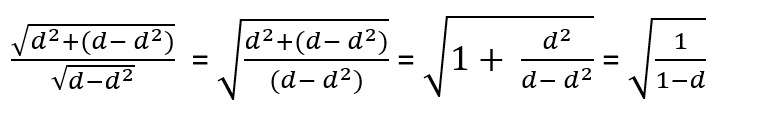or to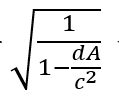where d now means simply the astronomical distance, as A = c^2/L.

Dividing the two gives the ratio of Azimuthal radius to internal Glome radius so the ‘Lensing’ shown means the degree to which the object under observation at some distance d/L gets ‘spread out’, suggesting that we could multiply this by the standard  factor for reduction of brightness with distance of 4pir^2

Explanation: Glomes (3-spheres or 4-balls) have an unobservable 4th dimensional radius of curvature.

The vertical axis represents the real distance to L,  but along it every point d on it has a corresponding point on the unobservable 4th dimension axis corresponding to sqrt(d-d^2) for its unobservable radius of 4th dimensional Glome curvature, and to sqrt(d^2 +(d-d^2)) for its unobservable radius of 4th dimensional Azimuthal curvature.

The ratio of these unobservable sqrt(d^2 +(d-d^2)) / sqrt(d-d^2), gives a third unobservable sqrt(1/(1-d)), which we can render into an observable by multiplying it by the expected drop off in brightness for distance d.

Prediction: The curve begins with a gentle almost flat slope, and this looks, the same as the results obtained for the type 1A supernovae. On the basis of the angle of the slope the Hubble constant became adjusted and the assumption of dark energy became adopted.

However, we cannot easily observe type 1As at huge distances, and the curve turns from a gentle slope into a steep curve at huge distances.

This hypothesis therefore predicts that at huge distances the dimming will become much more acute and that dark energy does not exist.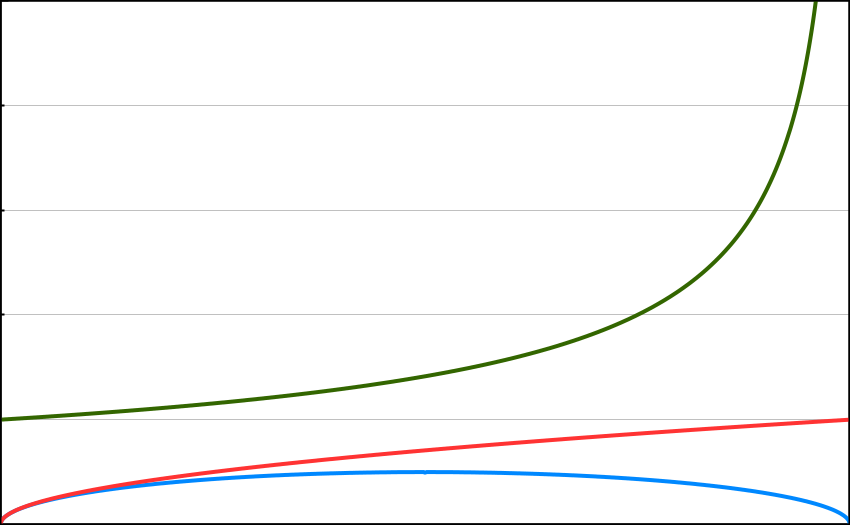Horizontal scale distance, Observer to antipode L.

Vertical scale, for red and blue lines, the unobservable 4th dimension of curvature radius. Green = hyperspherical lensing, corresponding to the increasing observed magnitude with distance d.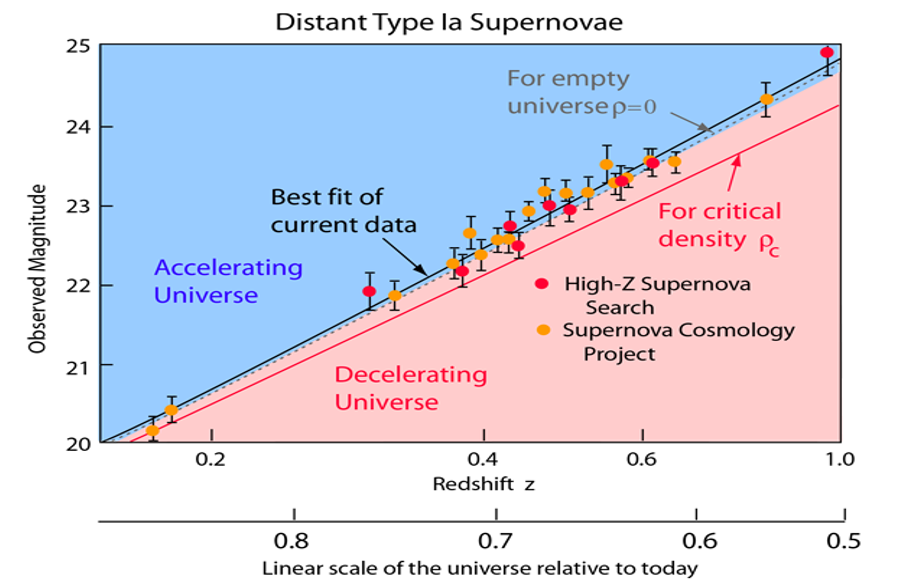Interpretation: This hypothesis forms part of the Hypersphere Cosmology hypothesis which models the universe as a Vorticitating (4-rotating) Hypersphere (Glome) which will appear to inside observers in Azimuthal Projection. This hypothesis also eliminates singularities, the big bang, inflation, and dark matter.

The treatment of gravity / spacetime curvature as a 4th dimension orthogonal to three-dimensional space and to time implies the non-quantisation of gravity and suggests that unification may lie in the geometrication of the quanta, perhaps in higher dimensions.

Read 607 times Last modified on Saturday, 20 April 2019 07:42
More in this category: « A Glome in Azimuth. Mayblog »